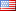English

Using solar cell panels to generate electricity for use with various devices. That must be considered is Use of solar panels sufficient for the amount of use. Electricity each day

Using solar cell panels to generate electricity for use with various devices. That must be considered is Use of solar panels sufficient for the amount of use. Electricity each day And the amount of temporary electricity usage each day In order to make predictions The total number of solar panels that must be used Example of daily electricity usage estimates Various electrical appliances Will indicate the electricity consumption of that electrical appliance In the form of electric power in watts (W), we can see the electrical power of that appliance. From the electrical label on the appliance or inside the manual of that appliance Such as long-lasting fluorescent lamps Will have a power of 40 watts, short of 20 watts. A 14-inch color television will have a power of about 70 watts (see the label after the device) after knowing the power in watts of various electrical devices Next is an estimate of the number of hours that are expected to be used per day, for example, and the value of electric power in watts of that electrical appliance multiplied by the number of hours used will be the power used within that hour, such as a 40-watt bulb. Use 3 hours a day = 40x3 = 120 watts (shows that If using 40 watts of electricity, 1 hour will consume 40 watts of power and if 3 hours will consume 120 watts of electricity.) 20 watts of electricity require 4 hours per day = 20x4 = 80 watts. 70-watt color television is enabled for 5 hours per day. = 70x5 = 350 watts. Therefore, the total electricity consumption is 120 + 80 + 350 = 550 watts. Therefore, the solar panel used must produce more than or equal 550 watts in order to be sufficient for use.